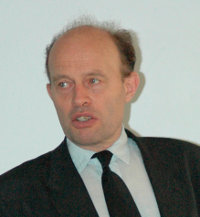Professor Gilbert De Mey from the Department of Electronics, University of Ghent, has just given a lecture at Ashurst Lodge on “The Minimum Energy for a one bit completion: A ‘proof’ of the Landauer Limit”.

The idea of how to compute the efficiency of electronic components is based on thermodynamic principles. Can a computer convert electric energy to information energy consuming a minimum amount of power?

Computers produce heat in order to perform these operations. In 2008, one bit operation required 1x10 -15J. In 2020 it is expected to be around 1x10-18J which is progressing towards the limit postulated by Landauer of 1x10-21J. This was predicted as early as 1961.

Boltzman developed a formula for entropy S, based on the k; Boltzman’s constant and the number of possibilities. We have for one bit operation W = 2 and hence, S = k ln 2. We can also write the amount of heat Q based on S and the absolute temperature, ie:

Q = T. S = k T ln 2

This amount of heat is the theoretical limit. It can only be avoided if reversible computing is used.

If the computer grows in size then energy consumption becomes a major problem. One solution is to make the process adiabatic. The process can be considered as adiabatic if the charging and uncharging is carried out slowly. This reduces the amount of energy converted to heat and the waste.

The price to pay is that the process is slower than with conventional computing. The solution is to publicise the operations and in that way, savings as high as 10 times can be achieved.

This is a new type of research, applying thermodynamics to information.